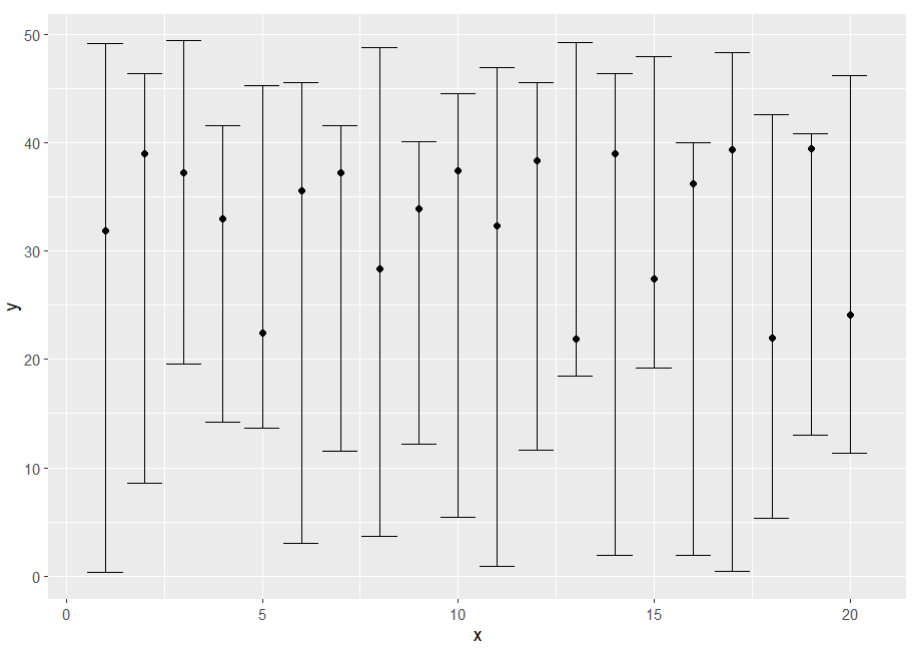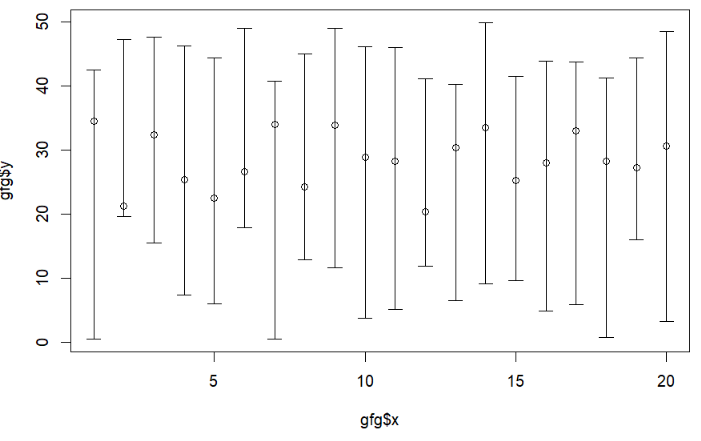# How to Plot a Confidence Interval in R?

• Last Updated : 19 Dec, 2021

In this article, we will discuss how to plot confidence intervals in the R programming language.

## Method 1: Plotting the confidence Interval using geom_point and geom_errorbar

In this method to plot a confidence interval, the user needs to install and import the ggplot2 package in the working r console, here the ggplot2 package is responsible to plot the ggplot2 plot and give the use of the package functionality to the users. Then the user needs to call the geom_point() function with the required parameters, which will be simply plotting the ggplot plot of the given data, and then the user has to call the geom_errorbar() function with the required parameters into it to get the confidence intervals which will be the error bars of the data passed in the R programming language.

To install and import the ggplot2 package in the R console, the user must follow the below syntax:

```install.packages("ggplot2")
library("ggplot2")```
• geom_point() function: This function uses the to point geom is used to create scatterplots.

Syntax: geom_point(mapping = NULL, data = NULL, stat = “identity”, position = “identity”, …)

Parameters:

• mapping: Set of aesthetic mappings created by aes() or aes_().
• data: The data to be displayed in this layer.
• stat: The statistical transformation to use on the data for this layer, as a string.
• position: Position adjustment, either as a string, or the result of a call to a position adjustment function.
• …: Other arguments passed.
• geom_errorbar() function: This function is used to plot the error bar of the given data.

Syntax: geom_errorbar(mapping = NULL, data = NULL,stat = “identity”, position = “identity”, …)

Parameters:

• mapping: The aesthetic mapping, usually constructed with aes or aes_string.
• data: A layer-specific dataset – only needed if you want to override the plot defaults.
• stat: The statistical transformation to use on the data for this layer.
• position: The position adjustment to use for overlapping points on this layer
• …: other arguments passed on to layer.

Example: Here, we will be using the geom_point() function to plot the points on the ggplot and then will be using the geom_errorbar() function with it to get the confidence intervals to the plot in the R programming language.

## R

 `# Import ggplot2 library ` `library``(``"ggplot2"``) ` ` `  `# Creating Data ` `gfg<-``round``(``data.frame``(x = 1:20, ` `                      ``y = ``runif``(20, 20, 40), ` `                      ``low = ``runif``(20, 0, 20), ` `                      ``up = ``runif``(20, 40, 50)), 4) ` ` `  `# Creating scatter plot with its ` `# confindence intervals ` `ggplot``(gfg, ``aes``(x, y)) + ``geom_point``() +  ` `geom_errorbar``(``aes``(ymin = low, ymax = up))`

Output:## Method 2: Plotting the confidence intervals using plotCI() function

In this method to plot the confidence intervals, the user needs to install and import the plotrix package to use its functionalities in the working R console, and then the user needs to call the plotCI() function with the data as the parameters of the function and further its function will directly plot the plot with the confidence intervals included in it in the R programming language.

To install and import the ggplot2 package in the R console, the user must follow the below syntax:

```install.packages("plotrix")
library("plotrix")```
• plotCI function: Given a set of x and y values and interval width or upper and lower bounds, plot the points with error bars.

Syntax: plotCI(x, y = NULL,ui, li, err=’y’, … )

Parameters:

• x,y: Coordinates for the center of error bars. y defaults to 1:n.
• UI: upper end of error bars.
• li: lower end of error bars.
• …: other plotting parameters.

Example: In this example, we will be using the plotCI() function to plot the confidence intervals in the plot of the given data in the R programming language.

## R

 `# Import plotrix library ` `library``(``"plotrix"``) ` ` `  `# Create Data ` `gfg<-``round``(``data.frame``(x = 1:20, ` `                      ``y = ``runif``(20, 20, 40), ` `                      ``low = ``runif``(20, 0, 20), ` `                      ``up = ``runif``(20, 40, 50)), 4) ` ` `  `# Create plotrix plot with confidence intervals ` `plotCI``(x = gfg\$x,y = gfg\$y,li = gfg\$low,ui = gfg\$up)`

Output:My Personal Notes arrow_drop_up
Recommended Articles
Page :